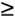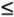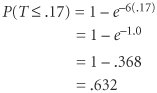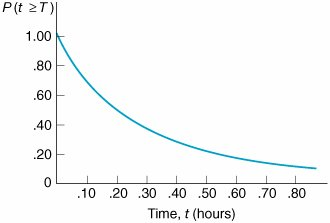# The Exponential Distribution

[Page 780]

The formula for the exponential distribution is

f ( t ) = µ e µ t , twhere

µ = average number of customers served during a specified period of time

t = service time

e = 2.71828

The probability that a customer will be served within a specified time period can be determined by using the exponential distribution, in the following form:

P ( Tt ) = 1 e µ t

If, for example, the service rate is six customers per hour, then the probability that a customer will be served within 10 minutes (.17 hour ) is determined as follows :Thus, the probability of a customer's being served within 10 minutes is .632. Figure C.2 is the exponential probability distribution for this service rate ( µ = 6).

##### Figure C.2. Exponential distribution for µ = 6Introduction to Management Science (10th Edition)
ISBN: 0136064361
EAN: 2147483647
Year: 2006
Pages: 358

Similar book on Amazon

flylib.com © 2008-2017.
If you may any questions please contact us: flylib@qtcs.net